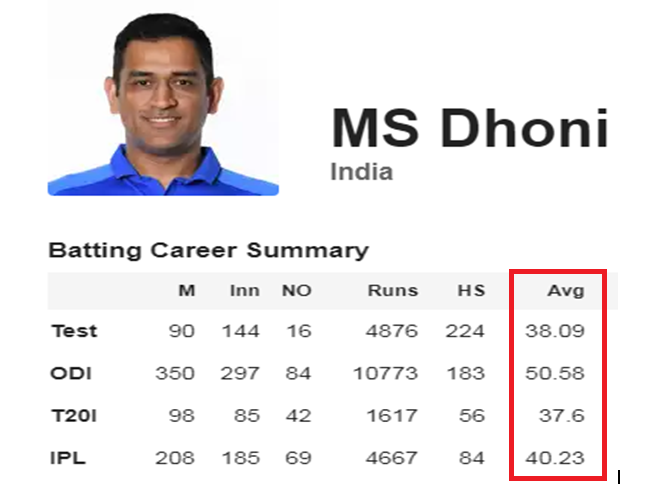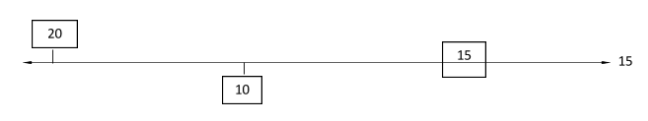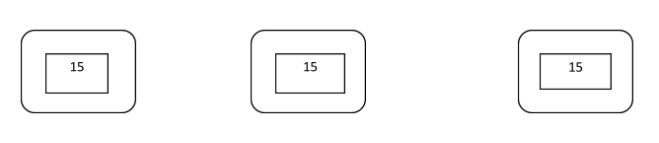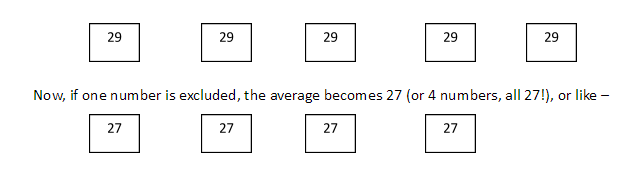Top

# Averages

Aptitude (Numeracy & Reasoning)- 21st century literacies

Average

Lesson Plan

Objective:

• Participants will get to understand basic concepts of average and its practical usage. This session would enable them to analyze the deviations of individual data from the mean of the given set under observation.

• To enable learners to solve questions of any kind related to average. Students will be able to deduce solutions and develop an ability to understand the concept of averages and its practical applications in daily life.

Total Time: 90 Minutes

Instructor Note:

This unit would deal with the basic nuances of the ever-important topic average. Owing to its importance, it becomes inevitable to form a strong foundation and holistic learning of the   same. Learners get to understand basic concepts of average and its practical usage. It would enable them to analyze the deviations of individual data from the mean of the given set under observation. Trainer is required to deliver this unit under an engaging environment by using practical examples and competitive activities.

(After concept discussion & practice) Display/distribute handout consisting of questions. Foundation section will compromise 4 questions, instruct students that they will be given 8 minutes to discuss among themselves & solve these 4 questions, whichever group solves maximum problems wins maximum points on the points table (refer opening protocol). Award 5 marks for each correct answer given by a group. Move around the class to check procedures used by students to solve problems. Once time is up, provide solutions and explanations for each question.

Switch to moderate level. This section comprises 3 questions and the cumulative time allotted will be 10 minutes. All other instructions remain the same.

Switch to HOTS. This section comprises 3 questions and cumulative time allotted will be 15 minutes. All other instructions remain the same.

Opening Protocol:

• Greet the participants.

• Take attendance.

• Form groups of participants with each group comprising 4 members. (For entire session)

• Draw points table on board and ask participants to name their group with some fancy names like “Aptitude thunders”, “Knights of Mathematics”, “Fantastic Statisticians” etc.

• Inform the participants that the group which tops the points table will be rewarded at the end of session.

• Recap the previous session by asking 1-2 participants to share with the class what they learnt and did in the previous session.

3

Minutes

Opening Activity:

• Alright everyone! Let’s discuss some unique approaches to calculate average, trust me you will fall in love with calculating averages.

• Before we begin, Look at the image below. Do you recognize him? Tell me something about him? (Quickly get a few answers from class), Now I want you to look at his stats covered in the red box which indicates average runs scored by MSD in respective formats.• Now, you have 10 minutes. In groups of 4, each participant has to choose one format and deduce the procedure for reaching the above-mentioned average in their chosen format. You are allowed to help each other.

• Now, one representative from each group has to come and share their calculation on any format (TEST/ODI/T20I/IPL) they wish to and discuss what average reflects about a batsman.

Trainer’s role:

• Award points to each group according to their calculations and definitions (Perfect or close). Consider step marking and close definitions.

• Explain the calculation and definition.

15

Minutes

Section 1:

• Let’s understand the concept of averages with an example.

• Case I: If there are three rooms such that A has 20 students, B has 10 students and C has 15 students.

 20 10 15

Then the average number of students in the rooms is calculated as [(20+10+15)/3] = 15.

So, average refers to the sum of observations divided by the number of observations.

We got 15 as the average number of students in three rooms, what does that mean?  What is “15” trying to say? Let’s have a look.

We had three numbers (20,10,15) whose average is 15. Let’s say below is a line representing “15” (Average value).In this figure you can see that 20 is above the average, 10 is below the average and 15 is on the average line. Let us see how much deviation 20 & 10 has from the average number.

• 20 has +5 deviation (Positive deviation)

• 10 has -5 deviation (negative deviation)

Net deviation = Positive deviation + Negative deviation i.e. [(+5) + (-5)] =0

Oh! Did we get a zero? Isn’t that interesting? Yes, it is! Average of an observation is such a number which equals the net deviation of the whole observation to “zero”.

So, if there is no deviation in any of the numbers in an observation, then what does that mean? It means that the whole set of observations has the same value. So, in the above case when you got 15 as the average number of students in three rooms, you can consider that each room has 15 students. This statement can also be represented as:Now, if I tell you to calculate the sum of observations in the above figure, what will you do?

Since you have to sum 15 three times so you will directly write 15*3=45 or you can rearrange this equation to 15=45/3. Can you observe this equation? This is exactly what you write as formula:

Average = Sum of observations/total number of observations

Now did you get an idea that if you know the concept, there is no need to memorize formulas because ultimately these formulas were derived from the concepts. Also, average has many practical applications in our daily life, so if we understand the concept, we can utilize them.

Section 2:

• Let’s understand the approach towards solving a problem on average.

• Case 2: The average of five numbers is 29. If one number is excluded, the average becomes 27. What is the excluded number?

Solution: According to the question, the average of five numbers is 29. Now, using the concepts learnt above, Let’s suppose that the value of all five numbers is 29 as shown in the figure below.Now in the second arrangement, the numbers become 27. Originally, they                      were 29, now how do we calculate the lost/excluded number?

Here is our observation: Looking at both the figures we can clearly deduce that initially we had five boxes with value 29 and now we have four boxes with value 27, so we have clearly lost a number/box with value “29”. But Is 29 my excluded number? No, try to find other changes between two arrangements.

Can you see that apart from one missing box of value “29”, now every box has lost two units each. So, total number of units lost from all the four remaining boxes will be 4*2=8. So, now you can say that the total number excluded/lost is 29+8=37.

What is the textual method to solve the above problem by using formulas?

Average of five numbers =29, Let us consider five numbers be A, B, C, D, E. Now according to the formula, Average= Sum of observations/Total number of observations. Applying formula, we can write (A+B+C+D+E)/5=29 or A+B+C+D+E= 145—Eq-1, Since one number is excluded, we can write (A+B+C+D)/4=27 or A+B+C+D=108— Eq-2. Putting value of Eq-2 in Eq-1 we get A= (145-108) = 37.

Now, tell me, is it required to perform the above mathematical operation to reach a solution? Leave back your traditional approaches and start visualizing these problems. This will help you solve real life problems based on averages quickly without using pen & paper.

Section 3:

• How to visualize solutions to a problem related to average? Let’s solve a problem to learn this.

• Case 3: The average weight of the teacher and six students is 12 kg which is reduced by 5 kg if the weight of the teacher is excluded. How much does the teacher weigh?

Solution: Just visualize along. There are 7 people whose average weight is 12 kg, so we can consider that each of them weighs 12 kg. If the weight of the teacher is excluded, the average weight is reduced by 5 kg, so now there are 6 people who weigh 7 kg each. Now, let’s calculate total weight loss= [12 kg (teacher’s weight which was excluded)] + [30kg (5kgs each of 6 students)]. So, the excluded weight was 30+12=42 kg and whose weight was excluded? It was the teacher. Thus, the teacher weighed 42 kg.

25

Minutes

 Section 4: Foundation The average of runs of a cricket player of 10 innings was 32. How many runs must he score in his next innings so as to increase his average of runs by 4? The average salary of 15 persons is Rs.5500. If the salary of one person is added, the average increases to Rs.5700. What is the salary of this one person? The average weight of a group of 20 boys was calculated to be 89.4 kg and it was later discovered that one weight was misread as 78kg instead of the correct one of 87kg. The correct average weight is? A class has 20 boys and 30 girls. The average age of boys is 11 years and that of girls is 12 years. What is the average age of the whole class? Section 5: Moderate The mean weight of 150 students in a class is 60 kg. The mean weight of boys is 70kg and that of girls is 55kg. How many boys are there in the class? The average marks of 120 students are 35. If the average of passed students was 39 and failed students was 15, then Find the number of students who have passed? The average age of 5 sisters is 20 year. If the age of the youngest sister is 4 year, what was the average age of the group of sisters at the birth of the youngest sister? Section 6: HOTS – HIGH ORDER THINKING SKILLS A cricketer gives 12.4 runs per wicket. He gives 26 runs and takes 5 wicket. He gave 26 runs and takes 5 wicket in a match after which his average becomes 12 runs per wicket. How many wickets had been taken till the last match? The average age of 8 members of a group is 40 years. The age of 1 member is 55 years. After his retirement a new member comes in his place of age 39.What is the effect of his retirement on the average age of group? 19 persons went to a hotel for a combined dinner party. 13 of them spent Rs.79 each on their dinner and the rest spent Rs.4 more than the average expenditure of all the 19. What was the total money spent by them? 8 minutes Answers (1) 76 (2) 8700 (3) 89.85 (4) 11.6 10 minutes Answers (1) 50 (2) 100 (3) 20 15 minutes Answers (1) 85 (2) 2 (3) 1536.07 EXPLANATION 10 Minutes Closing activity: Thank you everyone! Now, all of you are clear with your concepts of average. You will be getting materials for practice that you have to solve by yourself at home and bring doubts, if any. You will be provided handouts with concepts discussed which will help you brush up and revive your concepts any time in future. Trainer needs to calculate total points accumulated on the points table and reward the winning group. In case of a tie between two or more groups, reward each of the winning groups. Okay, Students! We will be ending our session with a joke. Let’s see how many of you get this. Three statisticians went out hunting, and came across a large deer. The first statistician fired, but missed, by a meter to the left. The second statistician fired, but also missed, by a meter to the right. The third statistician didn’t fire, but shouted in triumph, “On an average we got it”!! 1 Minutes Closing Protocol:  Thank the participants for their time. Announce the next time you will be visiting them. Share the topic of the next session. Share the names of reward-winning students with nodal officers and institution heads after the session. Post-session